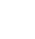# Richard Specification Compliance

``` Sub Add_matrix_to_Richards_Specification_sheets 'Adding the Specification Compliance scoring system for Richard 'Each sheet ending in Specification Compliance needs this applied 'but may be different sizes, numbers of rows```

``` 'Loop through all worksheets, and if name ends in Specification Compliance then run the loop Dim WS_Count As Integer Dim I As Integer WS_Count = ActiveWorkbook.Worksheets.Count For I = 1 To WS_Count ' Insert your code here. Worksheets(I).Select x = Right(ActiveSheet.Name, 25) 'If this is the worksheet were looking for, do some stuff If x = "Specificiation Compliance" Then 'Add the stuff in by finding start and end point With ActiveSheet lastrow = .Cells(.Rows.Count, "A").End(xlUp).Row End With 'Ensure rogue spaces are dealt with in the header were looking for Columns("A:A").Select Selection.Replace What:="Specification Headings ", Replacement:= _ "Specification Headings", LookAt:=xlPart, SearchOrder:=xlByRows, MatchCase _ :=False, SearchFormat:=False, ReplaceFormat:=False 'Match "Specification Header" then go down 1 for titles then 1 for formulas start_point = WorksheetFunction.Match("Specification Headings", ActiveSheet.Range("A:A"), 0) + 1 end_point = lastrow + 1 Range("F" & start_point) = "Mark / 10" Range("G" & start_point) = "Weighting" Range("H" & start_point) = "Mark" Range("F" & start_point & ":H" & end_point - 1).Select ' Selection.Borders(xlDiagonalDown).LineStyle = xlNone ' Selection.Borders(xlDiagonalUp).LineStyle = xlNone With Selection.Borders(xlEdgeLeft) .LineStyle = xlContinuous .ColorIndex = 0 .TintAndShade = 0 .Weight = xlThin End With With Selection.Borders(xlEdgeTop) .LineStyle = xlContinuous .ColorIndex = 0 .TintAndShade = 0 .Weight = xlThin End With With Selection.Borders(xlEdgeBottom) .LineStyle = xlContinuous .ColorIndex = 0 .TintAndShade = 0 .Weight = xlThin End With With Selection.Borders(xlEdgeRight) .LineStyle = xlContinuous .ColorIndex = 0 .TintAndShade = 0 .Weight = xlThin End With With Selection.Borders(xlInsideVertical) .LineStyle = xlContinuous .ColorIndex = 0 .TintAndShade = 0 .Weight = xlThin End With With Selection.Borders(xlInsideHorizontal) .LineStyle = xlContinuous .ColorIndex = 0 .TintAndShade = 0 .Weight = xlThin End With 'Loop down column G and add a formula For r = start_point + 1 To end_point - 1 ActiveSheet.Range("G" & r).Formula = "=E" & r & "/10" ActiveSheet.Range("G" & r).NumberFormat = "0.00" ActiveSheet.Range("H" & r).Formula = "=F" & r & "*G" & r ActiveSheet.Range("H" & r).NumberFormat = "0.00" Next r 'Put the sum field and format into the page ActiveSheet.Range("H" & end_point).Formula = "=Sum(H" & start_point + 1 & ":H" & end_point - 1 & ")" ActiveSheet.Range("H" & end_point).Interior.Color = 12611584 ActiveSheet.Range("H" & end_point).Font.Color = 16777215 Range("H" & end_point).Select ' Selection.Borders(xlDiagonalDown).LineStyle = xlNone ' Selection.Borders(xlDiagonalUp).LineStyle = xlNone With Selection.Borders(xlEdgeLeft) .LineStyle = xlContinuous .ColorIndex = 0 .TintAndShade = 0 .Weight = xlThin End With With Selection.Borders(xlEdgeTop) .LineStyle = xlContinuous .ColorIndex = 0 .TintAndShade = 0 .Weight = xlThin End With With Selection.Borders(xlEdgeBottom) .LineStyle = xlContinuous .ColorIndex = 0 .TintAndShade = 0 .Weight = xlThin End With With Selection.Borders(xlEdgeRight) .LineStyle = xlContinuous .ColorIndex = 0 .TintAndShade = 0 .Weight = xlThin End With With Selection.Borders(xlInsideVertical) .LineStyle = xlContinuous .ColorIndex = 0 .TintAndShade = 0 .Weight = xlThin End With With Selection.Borders(xlInsideHorizontal) .LineStyle = xlContinuous .ColorIndex = 0 .TintAndShade = 0 .Weight = xlThin End With '***** End If Next I '***** 'This is where the code for applying the scoring matrix into the Specification Compliance tab ends ```

```End Sub ```